/ /

How to Perform Form Calculation in the Input Table Field?

# How to Perform Form Calculation in the Input Table Field?

It is now possible to use the Input Table (Matrix) field to perform form calculation.

Here in our example, we will set the matrix field similar to a survey questionnaire with ratings or value on them, we will calculate the total rating using the Form Calculation widget. You can follow the steps below on how to do that.

In Version 4.0, we call the Matrix Table from Version 3.0, Input Table. It can be found in the Quick Elements section of the Basic tab when you add Form Elements to the form.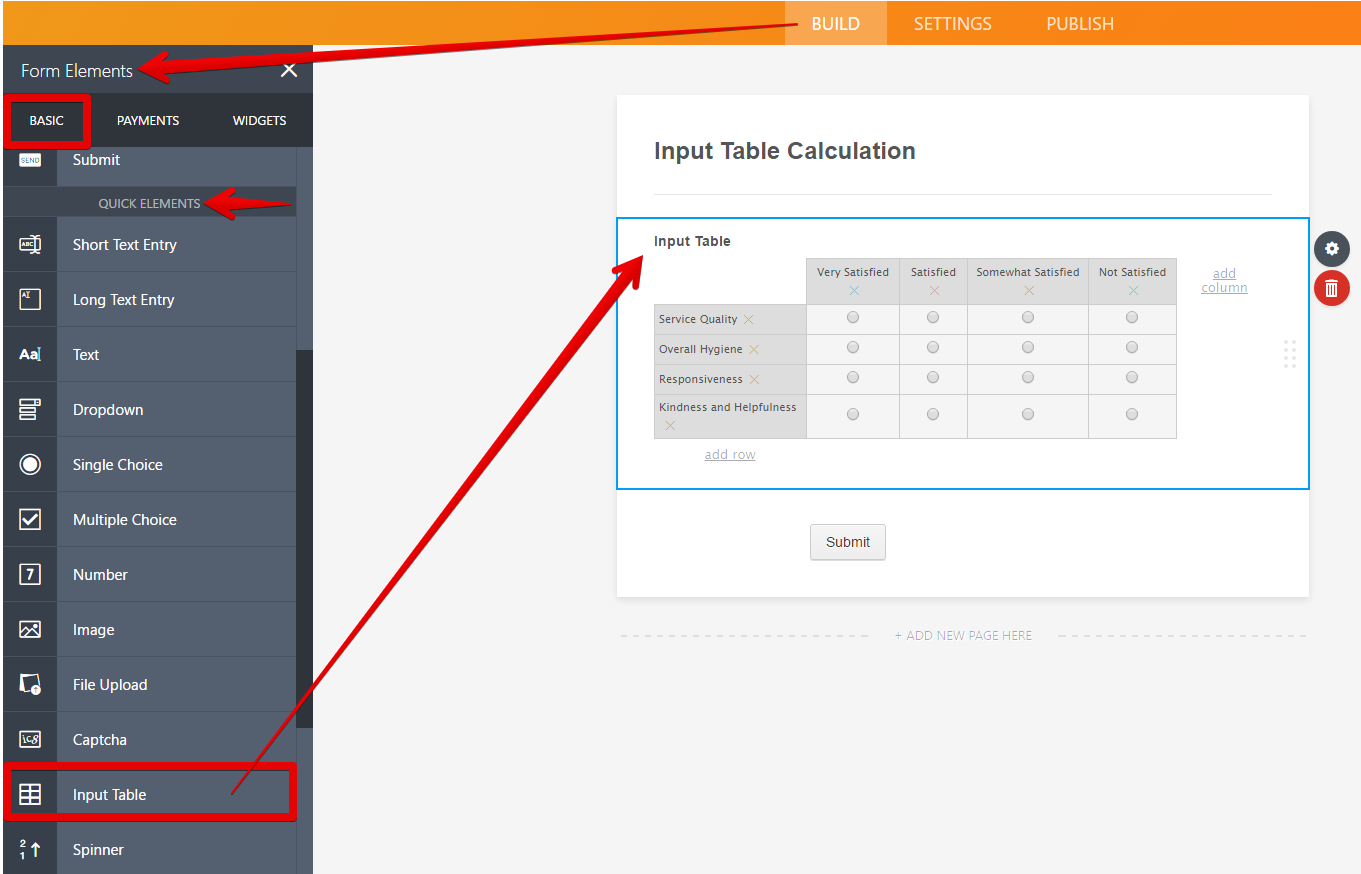Once you've added the Input Tableselect an input type from its properties (cogwheel icon). From the same Fields tab of the Input Table properties, you will be able to set row and column headers.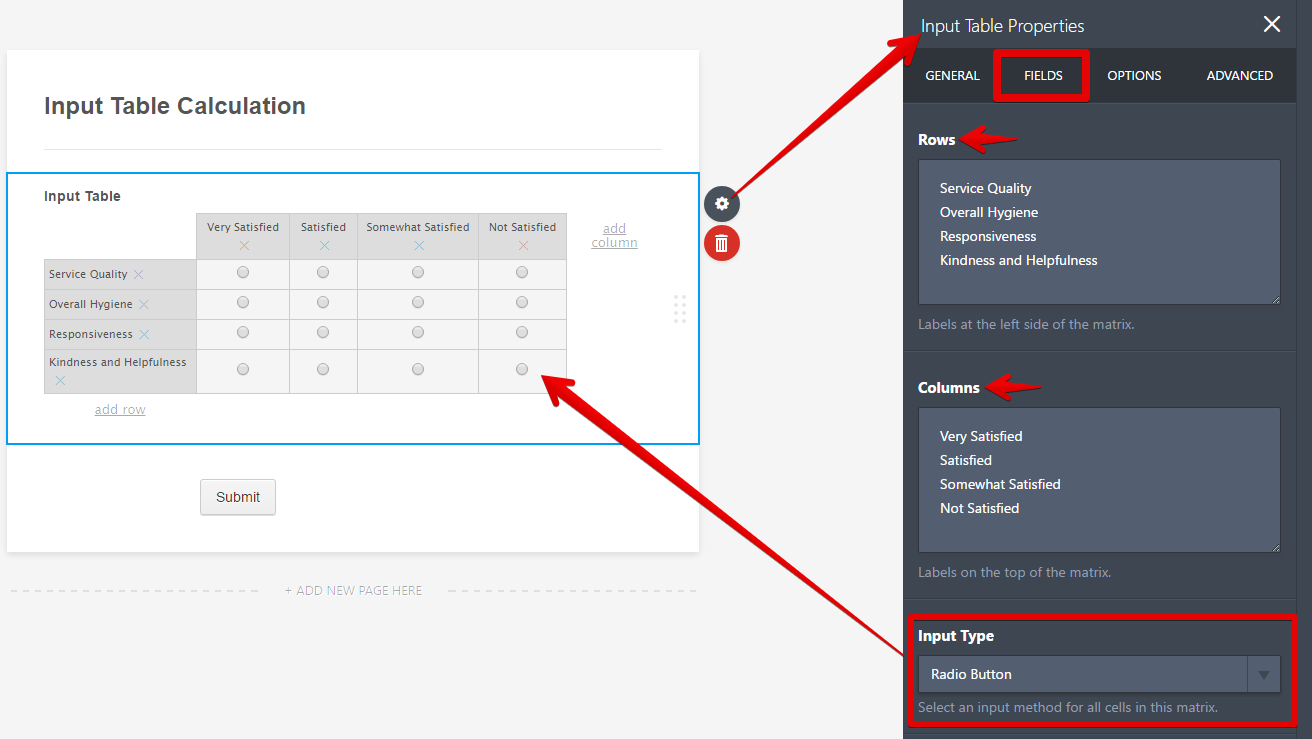After constructing the table, you now need to set calculation values for each of its cell (matrix sub-field). To do that, go to the Options tab and enter the values in the provided text area for calculation values following this simple rule, "Separate each column with a comma and each row with a new line."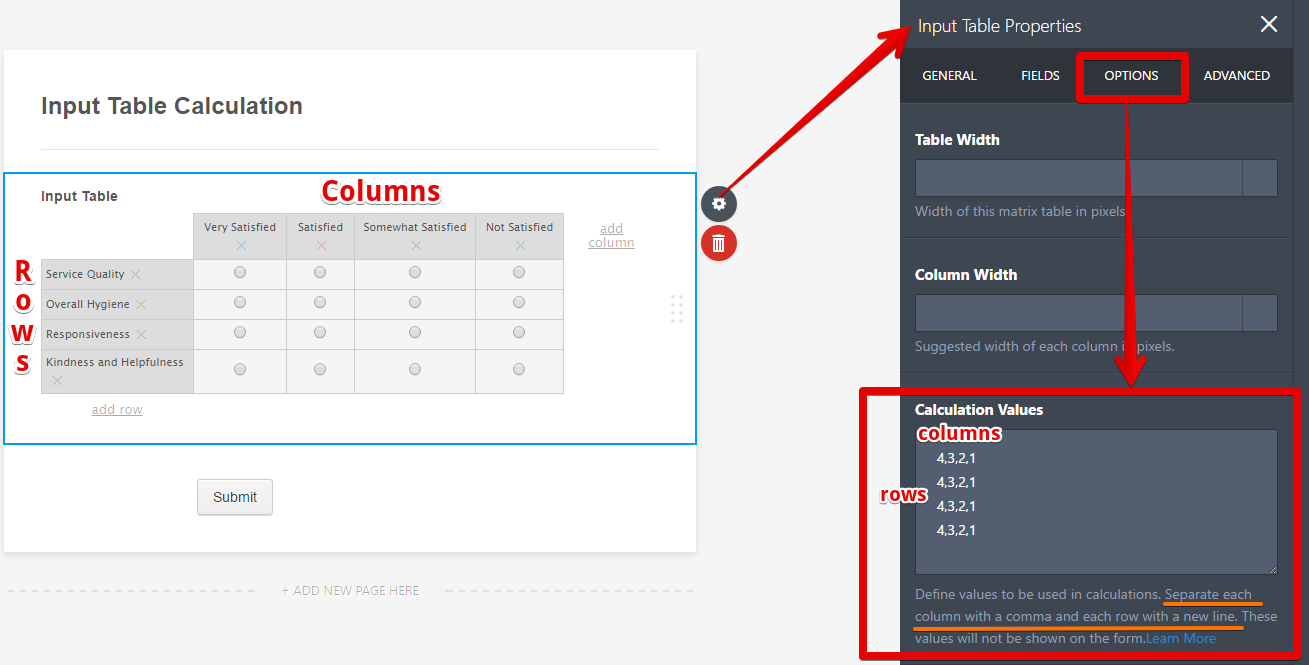In the example above, 4 Rows means 4 lines and 4 columns means 4 values separated by a comma.

Note: Choosing a different input type for the table, like a text box or number text box, if you want your respondents to define values for each cell, will not require you to set calculation values.

To get the total value of the selected cell(s) on the table, you will use the Form Calculation widget from the Widgets tab when you add Form Elements to the form.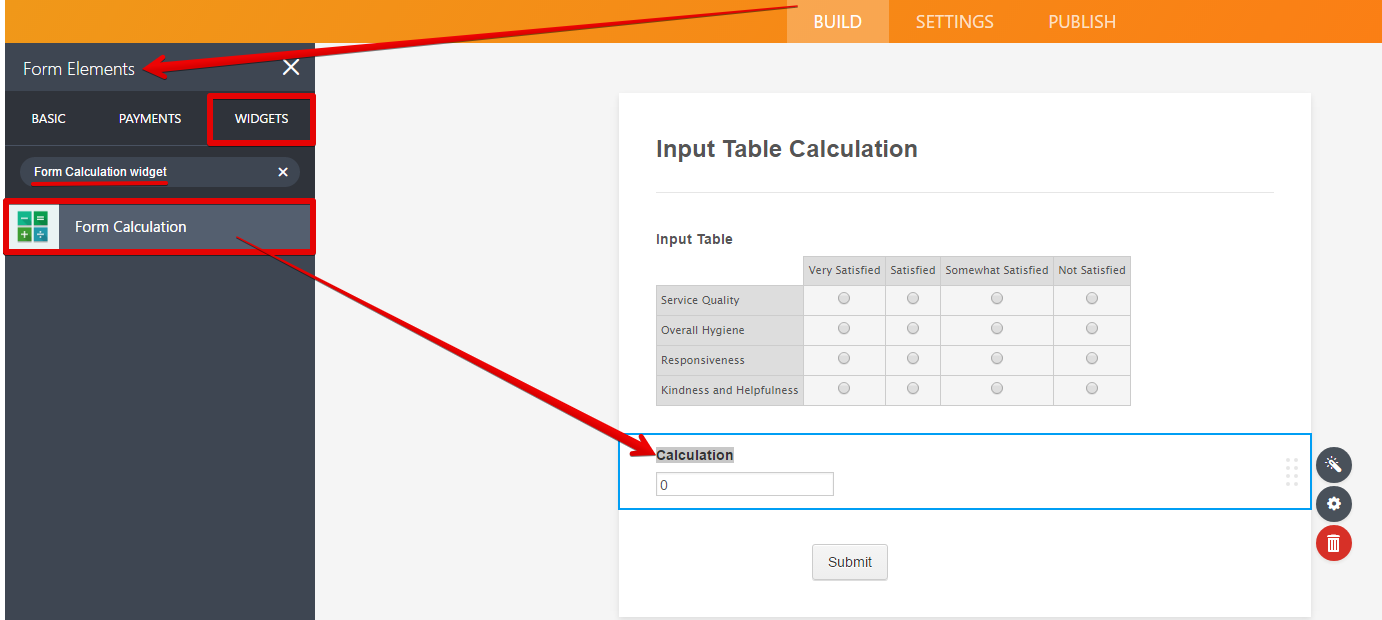To perform the calculation, you need to add the input table to the calculation area of the Form Calculation widget.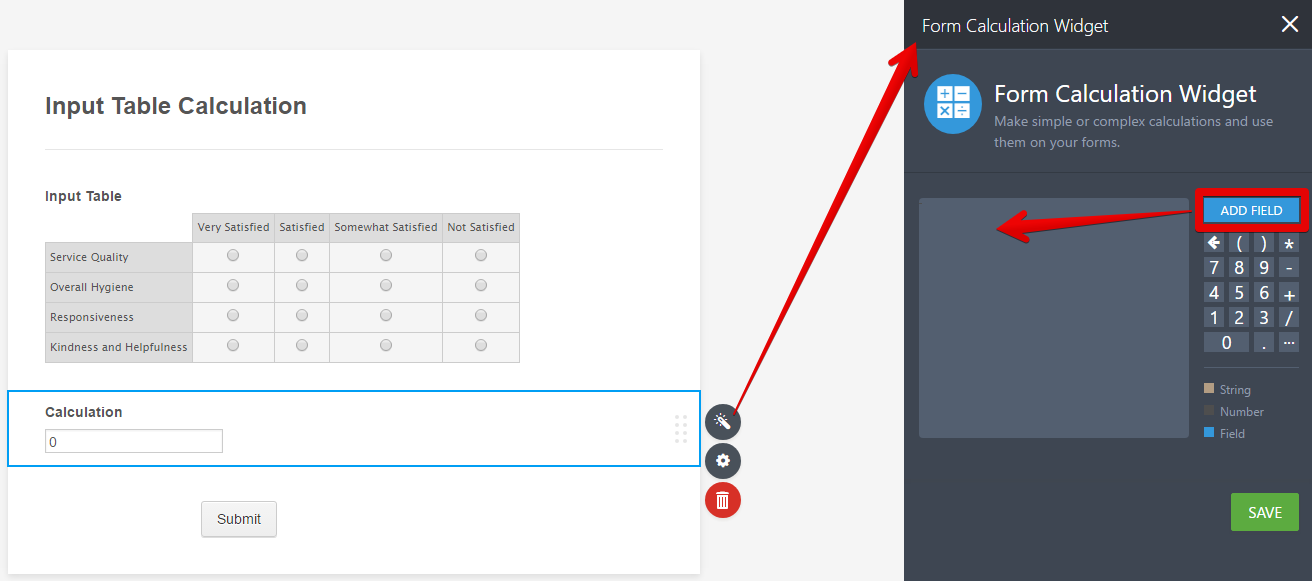Clicking the "Add Field" button shown in the screenshot above lets you add fields to the computation.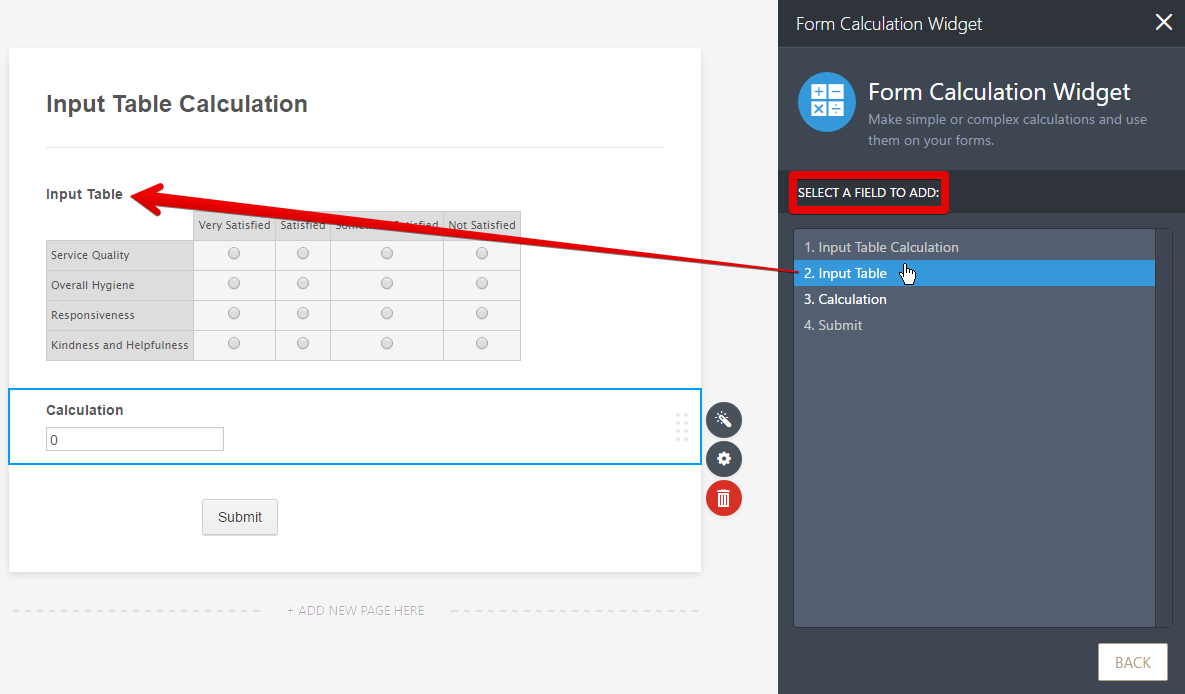Adding an Input Table field will let you select which row (radio button) or cell (checkbox field) in the table is to be included in the computation. (This is also referred as matrix sub-fields.)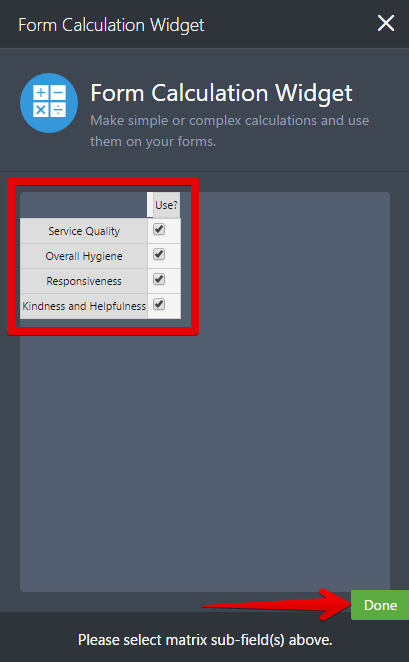After selecting the matrix sub-field(s) and clicking Done, you will see the selected sub-fields of the input table in the calculation area of the Form Calculation widget. From there, you will be able to create your own formula to get a total value. The calculation pad on the right will surely help you with the simple to complex mathematical tasks.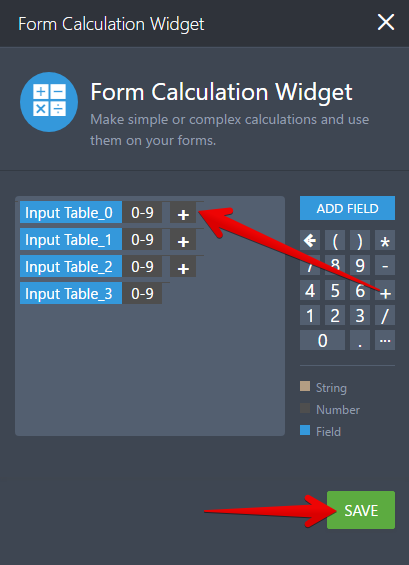Sample Form that you can also clone to your account for testing: https://www.jotform.com/form/70984576350970

Other references that might be helpful:

Form Calculation - Math Function Reference

You can check the sample form here: http://form.jotformpro.com/form/51691444490963?

Contact Support:
Our customer support team is available 24/7 and our average response time is between one to two hours.
Our team can be contacted via:
Contact JotForm Support: https://www.jotform.com/contact/

### Send Comment

•tasneem.waly

How can I hide the calculation widget from the form to everyone but me?
I want the calculation but don't want it to appear in the public form

•Hi,
Can I assign a default value to a specific cell of my input table?
I have set it to numeric data and I want one full column of cells to be equal to ‘1’ by default and accept manual changes.
Or if that cell is not filled up by the user then assign the number 1 in the calculation

•AAISmarketing

Is there a way to sum each column individually? I need the break down of each unique column totaled.... not a accumulation of the entire tables results.

•julietteprice

this is too complex, I wonder if I could pay for someone to insert a table for me?

•michael_pire

Bonjour, Comment mettre des nombres négatifs dans le calcul? Par exemple:
10,-5?

•Rdiz

Hello,

I´m trying to set a simple add formula to an input table but I need to use dropdown type and then add the value of the row based on the respond on the dropdown. I´m having trouble setting the formulas to each table.

Can you help me?

•kstover89

Hello there,

Is there a way to format this widget/table to hide empty fields/cells when the form is submitted. For example, I have 10 rows in my table but if the person submitting the form only fills out 3, can we create a way to hide the empty 7 from the form when it is sent through email?

•office.ojasnisarga

hi all i have one prob. in table entry i want to enter only alphabets and numbers i dont want extra character like , . / = Etc in form pls guide me.

•Nelson131

I am having issues with my forms calcualtions. It keeps generating random decimals.

•jspitz65

Is there a condition option for Equal To or Less Than?

•bakerandgrazeforms

How do I give monetary values to calculation values? Is it as simple as:

£3.00
£2.00
£5.00

When I use the calculation widget it only calculates the total quantities and not the total cost of the quantities?

•foziamhb

Hi,

I am creating a table for survey and all columns have to be selected by a click, whereas I want the last column to be typed in, incase if they have any suggestions. how can I do this? can you help me in this?

•DougSk

I want to assign a certain numerical value to each of the selections: no problem = 0, Very mild problem = 1, Mild or Slight Problem = 2, Moderate problem = 3, severe problem = 4, problem as bad as it gets = 5.

HOW?

•systemisedbydesign

is there a way we can use the cell input value for the calculation? eg: for a cell that collects a number for total hours worked, can we use that value rather than a static value that I apply if the field is filled?

•topprime

Hey guys, how can i make it responsible?

•topprime

Hey guys. I need to make one of the columns read-only, how can i do it?

•HuttonSafetyGroup

Hey guys! Now that you've implemented the Multi-Type Columns in the Input Table (YAY!), would you be able to do a page like this for how to do calculations based on answers with dropdowns, etc? I may be over thinking it but it seems quite complicated.

For instance, I want to be able to populate a "Describe below" box if someone selects an answer in one column that uses a dropdown, but also populate a different "Describe" box if they select a radio button answer, etc.

Thanks!

•jorgemanriquetc

Hi:
How can I get a different answer in each row along the matrix?
Thank you very much.

•SilverOakDM

I don't know how to word this question, but I can explain what I'm trying to accomplish.
In column one, players input their values. Each value has a modifier. I want to be able to type "12" in column 1 and have column 2 automatically spit out the correct modifier value (1). Is there a way for me to program this?

•justdawn

how do i make it that you can only select certain columns for each row
IE
row one- the only options can be column 1 and 3
row two the only options can be column 2 and 4

thanks

•aorca

How can I multiply qty and price= total and the whole total?

https://form.jotform.com/aorca/order-form

•Cmartin18

Hello,

How can this function be used using the "drop down" selection with more than one value can be selected?

•artnilsen

The answer to my comment below just gave me a link to this web page on which I had the "How to" question."
As I understand it, I do not need a calculation. I just need to be able to move the contents of a field previously entered into one or more of the rows and columns in a table. Please clarify your response.
Thank you.

•artnilsen

How can I set the value in a table cell (As an example: Row 1/Column 1) equal to a field entered above?

•sellyon

I have a table with 6 rows and 3 colums. The fields of the colums (radio buttoms) are : choice 1, choice 2, choice 3.
Is it possible to limit the form to only one choice 1, only one choice 2, olny one choice 3, in the table ?

Thank you

Michel

•elipass

Dear Developer, i need to find an axample of form calculation for money Eur and USD. For example like this:https://eu.jotform.com/build/82752707278365 but in the field cost i need Eur or USD (or other kind of valute), after in the cost total i need the total live of the cost that I have insert in the grid... I think very easy for you but i don't find any kind of form in template... Can you help me?? thanks so much. I need for give to person the possibility of calculate the cost easy and sign and send to the administration office. thanks for your help
best regards
stefano

•emaarproperties

for the emoji's the calculation is coming wrong for a 5 point scale..it still shows up with 7 points. what can we do to fix this?

•SysCloudUS

Hi - I am using radio buttons in a table and I want the person to be able to select multiple options in a row if they so choose - how can I do this?

•razana

i only have 1 row and 1 column, how to widen the column? tQ

•CSBRAGA

I'm trying to total a column using the Form Calculation Widget and I don't know what I'm doing wrong.
I can't seem to get the + sign after the added field and then the numbers are calculating higher than they actually are. I put in 10 and 10,000 is showing up in the calculation field.

•jimwhite

Am I able to have two separate calculations for one table? For example, I am using as a daily time sheet and have a column for regular hours worked (which is calculating correctly) and a column for overtime hours worked (which is only duplicating what is in the regular hours field). I am doing the formulas the exact same for each different column but not getting two separate answers.

•MummySOSLtd

Can I make it so that each drop down option can only be used once?

•FifteenAndFifteen

How can I delete a field and add a different field from the add field button?

•KPWM

I want to assign values to each question in my matrix, but I don't want the person filling out the form to see the value. I only want to be able to see it. Is this possible?

•lansingparty

I am hoping to get some help here because I am sure this is possible and I am simply missing how to do this. This is a limo company with a few variables. Lets make it simple and say we have limoA, limoB, and limoC. Each of these limos have an hourly rate for Weekday, Friday, Saturday. How would I set this up so the customer could fill out the form select the day of the week, use a check box form to select what they want quotes on, then depending on the hours selected multiply the value by the hours?

•ZacharyRichards

I wanted to know if it were possible to create a calculation in the actual matrix.
For example, a cell in the matrix equals a date that someone put in above the matrix.
Is this possible

•poudreriver

I am trying to count how many checkboxes are checked in a matrix.

It is the attendance sheet here: https://form.jotform.com/63495321047152

I am trying to figure out how I can set the value of each checkbox to 1. I know that I can insert 1, but I don't want that to show in the headers. If I hide the headers, then the months can't show. I need to be able to show the months across the top. Do you have any suggestions for how I an do this?

Thanks!

•aysaquing

Can I set condition on form matrix calculation? For example if column 5 as remarks says Best, the calculator will just compute all fields (rows) with best. Is that possible? Thanks.

•jannyfer.chua

Hi,  I have a set of 28 questions and 5 radio columns (Never, Rarely, Sometimes, Very often, Always).  a) How do I set values for each column and when they are selected, the values are added up? e.g. Never=1, Rarely=2, Sometimes=3, Veryoften=4, Always=5  if Never is selectedx2, rarely x1, always x2 = the total is 14.  b) How do I set the 28 questions to calculate 4 different totals from the above columns. eg. Qs 1-7, 8-14, 15-21, 22-28 and then set a formula to only show the results for the highest total?

•IDOEWebmaster

How do I multiply the value of column 1 and column 2 and show the result value in the column 3 of the matrix table?
Thanks.

•Communicaid

ok, thank you for your help

•Communicaid

Hello,
I've created a matrix question with dropdown list "yes" and "no" and I wwould like to add the values to the "yes"=1 and "no"=0.
my question is: can I use the same role live above : yes1?
what is the css code to hide the numbers?
•